## How to Calculate and Solve for True Stress | The Calculator Encyclopedia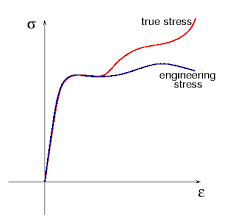The image above represents the true stress.

To compute for the true stress, two essential parameters are needed and these parameters are force (F) and instantaneous area (Ai).

The formula for calculating true stress:

σT = F / Ai

Where;
T = True Stress
F = Force
Ai = Instantaneous Area

Let’s solve an example;
Find the true stress when the instantaneous area is 60 with a force of 25.

This implies that;
F = Force = 25
Ai = Instantaneous Area = 60

σT = F / Ai
σT = 25 / 60
σT = 0.416

Therefore, the true stress is 0.416 Pa.

Calculating the Force when True Stress and Instantaneous Area is Given.

F = Ai x σT

Where;
F = Force
σT = True Stress
Ai = Instantaneous Area

Let’s solve an example;
Find the force when the instantaneous area is 30 with a true stress of 15.

This implies that;
σT = True Stress = 15
Ai = Instantaneous Area = 30

F = Ai x σT
F = 30 x 15
F = 450

Therefore, the force is 450.

## How to Calculate and Solve for Maximum Velocity to avoid Overturning of a Vehicle moving along a Level Circular Path | The Calculator Encyclopedia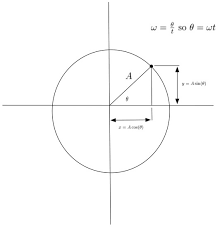The image above represents the maximum velocity to avoid overturning of a vehicle moving along a level circular path.

To compute for the maximum velocity, four essential parameters are needed and these parameters are Acceleration due to Gravity (g), Height of Centre of Gravity of the Vehicle from Ground Level (h), Radius of Circular Path (r) and Half of the Distance between the Centre Lines of the Wheel (a).

The formula for calculating the maximum velocity:

vmax = √(gra / h)

Where:
vmax = Maximum Velocity to avoid Overturning of a Vehicle moving along a Level Circular Path
g = Acceleration due to Gravity
h = Height of Centre of Gravity of the Vehicle from Ground Level
r = Radius of Circular Path
a = Half of the Distance between the Centre Lines of the Wheel

Let’s solve an example;
Find the maximum velocity when the Acceleration due to Gravity (g) is 10.2, Height of Centre of Gravity of the Vehicle from Ground Level (h) is 14, Radius of Circular Path (r) is 22 and Half of the Distance between the Centre Lines of the Wheel (a) is 32.

This implies that;
g = Acceleration due to Gravity = 10.2
h = Height of Centre of Gravity of the Vehicle from Ground Level = 14
r = Radius of Circular Path = 22
a = Half of the Distance between the Centre Lines of the Wheel = 32

vmax = √(gra / h)
vmax = √((10.2)(22)(32)/14)
vmax = √((7180.79)/14)
vmax = √(512.91)
vmax = 22.647

Therefore, the maximum velocity to avoid Overturning of a Vehicle moving along a Level Circular Path is 22.647 m/s.

## How to Calculate and Solve for the Reaction at the Inner Wheel of a Vehicle moving along a Level Circular Path | The Calculator Encyclopedia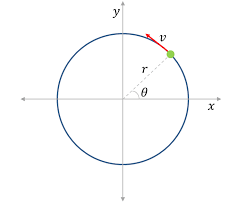The image represents reaction at the inner wheel of a vehicle moving along a level circular path.

To compute for the reaction, six essential parameters are needed and these parameters are Mass of the Vechicle (m), Acceleration due to Gravity (g), Velocity of the Vehicle (v), Height of Centre of Gravity of the Vehicle from Ground Level (h), Radius of Circular Path (r) and Half of the Distance between the Centre Lines of the Wheel (a).

The formula for calculating the reaction at the inner wheel of a vehicle moving along a level circular path:

RA = mg / 2[1 – v²h / gra]

Where:
RA = Reaction at the Inner Wheel of a Vehicle moving along a Level Circular Path
m = Mass of the Vechicle
g = Acceleration due to Gravity
v = Velocity of the Vehicle
h = Height of Centre of Gravity of the Vehicle from Ground Level
r = Radius of Circular Path
a = Half of the Distance between the Centre Lines of the Wheel

Let’s solve an example;
Find the reaction when Mass of the Vechicle (m) is 13, Acceleration due to Gravity (g) is 9.8, Velocity of the Vehicle (v) is 11, Height of Centre of Gravity of the Vehicle from Ground Level (h) is 5, Radius of Circular Path (r) is 7 and Half of the Distance between the Centre Lines of the Wheel (a) is 3.

This implies that;
m = Mass of the Vechicle = 13
g = Acceleration due to Gravity = 9.8
v = Velocity of the Vehicle = 11
h = Height of Centre of Gravity of the Vehicle from Ground Level = 5
r = Radius of Circular Path = 7
a = Half of the Distance between the Centre Lines of the Wheel = 3

RA = mg / 2[1 – v²h / gra]
RA = 13(9.8) / 2[1 – (11)²(5) / (9.8)(7)(3)]
RA = 127.4 / 2[1 – (121)(5) / 205.8]
RA = 63.7[1 – 605 / 205.8]
RA = 63.7[1 – 2.939]
RA = 63.7[-1.939]
RA = -123.56

Therefore, the reaction at the inner wheel of a vehicle moving along a level of circular path is -123.56 N.

## How to Calculate and Solve for Road Bank Angle, Velocity and Radius of a Body in Motion of Circular Path | The Calculator Encyclopedia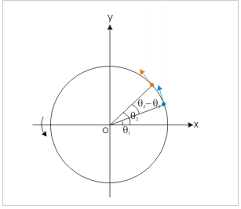The image represents road bank angle in circular motion.

To compute for the road bank angle, three essential parameters are needed and these parameters are velocity (v), acceleration due to gravity (g) and radius (r).

The formula for calculating the road bank angle;

θ = tan-1( / gr)

Where;
v = Velocity
g = Acceleration due to Gravity

Let’s solve an example;
Find the road bank angle where the acceleration due to gravity is 9.8, velocity is 35 and radius is 18.

This implies that;
v = Velocity = 35
g = Acceleration due to Gravity = 9.8

θ = tan-1( / gr)
θ = tan-1(35² / (9.8)(18))
θ = tan-1(1225 / 176.4)
θ = tan-1(6.94)
θ = 81.81°

Therefore, the road bank angle is 81.81°.

Calculating the Velocity when Road Bank Angle, Acceleration due to Gravity and Radius is Given.

v = √gr.tan θ

Where;
v = Velocity
g = Acceleration due to Gravity

Let’s solve an example;
Given that the road bank angle is 50, radius is 15 and acceleration due to gravity is 9.8. Find the velocity?

This implies that;
θ = Road Bank Angle = 50
g = Acceleration due to Gravity = 9.8

v = √gr.tan θ
v = √(9.8 x 15)(tan 50)
v = √(147)(1.1917)
v = √175.1799
v = 13.235

Therefore, the velocity is 13.235.

## How to Calculate and Solve for the Reaction at the Outer Wheel of a Vehicle moving along a Level Circular Path | Nickzom Calculator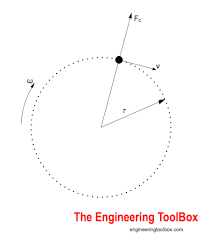The image represents reaction at the outer wheel of a vehicle moving along a level circular path.

To compute for the reaction, six essential parameters are needed and these parameters are Mass of the Vechicle (m), Acceleration due to Gravity (g), Velocity of the Vehicle (v), Height of Centre of Gravity of the Vehicle from Ground Level (h), Radius of Circular Path (r) and Half of the Distance between the Centre Lines of the Wheel (a).

The formula for calculating the reaction at the outer wheel of a vehicle moving along a level circular path:

RB = mg / 2[1 + v²h/gra]

Where;
RB = Reaction at the Outer Wheel of a Vehicle moving along a Level Circular Path
m = Mass of the Vechicle
g = Acceleration due to Gravity
v = Velocity of the Vehicle
h = Height of Centre of Gravity of the Vehicle from Ground Level
r = Radius of Circular Path
a = Half of the Distance between the Centre Lines of the Wheel

Let’s solve an example;
Find the reaction when Mass of the Vechicle (m) is 12, Acceleration due to Gravity (g) is 9.8, Velocity of the Vehicle (v) is 28, Height of Centre of Gravity of the Vehicle from Ground Level (h) is 16, Radius of Circular Path (r) is 8 and Half of the Distance between the Centre Lines of the Wheel (a) is 4.

This implies that;
m = Mass of the Vechicle = 12
g = Acceleration due to Gravity = 9.8
v = Velocity of the Vehicle = 28
h = Height of Centre of Gravity of the Vehicle from Ground Level = 16
r = Radius of Circular Path = 8
a = Half of the Distance between the Centre Lines of the Wheel = 4

RB = mg / 2[1 + v²h/gra]
RB = 12 x 9.8 / 2[1 + 28² x 16/9.8 x 8 x 4]
RB = 117.60 / 2[1 + 784 x 16/313.6]
RB = 58.80[1 + 40]
RB = 58.80
RB = 58.80
RB = 2410.8

Therefore, the reaction of the outer wheel of a vehicle moving along a level circular path is 2410.8 N.

## How to Calculate and Solve for Centripetal Acceleration, Angular Velocity and Radius of Circular Path of a Body | The Calculator EncyclopediaThe image above represents centripetal acceleration in a motion of circular path.

To compute for the centripetal acceleration, two essential parameters are needed and these parameters are angular velocity (ω) and radius of circular path (r).

The formula for the calculating centripetal acceleration:

a = ω²r

Where:
a = Centripetal Acceleration
ω = Angular Velocity
r = Radius of Circular Path

Let’s solve an example;
Find the centripetal acceleration with an angular velocity of 33 and a radius of 21.

This implies that;
ω = Angular Velocity = 33
r = Radius of Circular Path = 21

a = ω²r
a = 33² x 21
a = 1089 x 21
a = 22869

Therefore, the centripetal acceleration is 22869 m/s².

Calculating the Angular Velocity when Centripetal Acceleration and Radius of Circular path is Given.

ω = √a / r

Where:
ω = Angular Velocity
a = Centripetal Acceleration
r = Radius of Circular Path

Let’s solve an example;
Find the angular velocity with a centripetal acceleration of 320 and a radius of 8.

This implies that;
a = Centripetal Acceleration = 320
r = Radius of Circular Path = 8

ω = √a / r
ω = √320 / 8
ω = √40
ω = 6.32

Therefore, the angular velocity is 6.32.

## How to Calculate and Solve for Maximum Velocity, Coefficient of Friction and Radius of a Body in Motion of Circular Path | Nickzom Calculator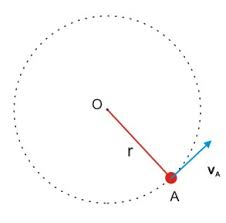The image above represents maximum velocity in circular motion.

To compute for the maximum velocity, three essential parameters are needed and these parameters are coefficient of friction (μ), radius (r) and acceleration due to gravity (g).

The formula for calculating maximum velocity:

Vmax = √(μgr)

Where;
Vmax = maximum velocity
μ = coefficient of friction
g = acceleration due to gravity

Let’s solve an example;
Find the maximum velocity when the coefficient of friction is 14 with a radius of 7 and acceleration due to gravity of 9.8.

This implies that;
μ = coefficient of friction = 14
g = acceleration due to gravity = 9.8

Vmax = √(μgr)
Vmax = √(14 x 7 x 9.8)
Vmax = √(960.40)
Vmax = 30.99

Therefore, the maximum velocity is 30.99 m/s.

Calculating the Coefficient of Friction when the Maximum Velocity, Radius and Acceleration due to Gravity is Given.

μ = Vmax2 / gr

Where;
μ = coefficient of friction
Vmax = maximum velocity
g = acceleration due to gravity

Let’s solve an example;
Find the coefficient of friction with a maximum velocity of 120, radius of 15 and acceleration due to gravity is 9.8?

This implies that;
Vmax = maximum velocity = 120
g = acceleration due to gravity = 9.8

μ = Vmax2 / gr
μ = 1202 / 15 x 9.8
μ = 14400 / 147
μ = 97.96

Therefore, the coefficient of friction is 97.96.

## How to Calculate and Solve for Superelevation, Guage of Track, Velocity and Radius of a Body in Circular Path Motion | The Calculator Encyclopedia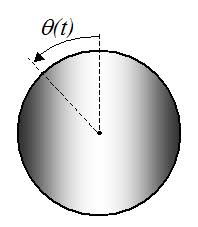The image above represents a body in superelevation.

To compute for the Superelevation, four essential parameters are needed and these parameters are Gauge of the track (G), velocity of the body (v), radius of the curve (r) and acceleration due to gravity (g).

The formula for calculating the circular path motion:

S = Gv² / gr

Where:
S = Superelevation
G = Gauge of the track
v = velocity of the body
r = radius of the curve
g = acceleration due to gravity

Let’s solve an example;
Find the circular path motion when Gauge of the track is 14, velocity of the track is 47, radius of the curve is 21 and acceleration due to gravity is 9.8.

This implies that;
G = Gauge of the track = 14
v = velocity of the body = 47
r = radius of the curve = 21
g = acceleration due to gravity = 9.8

S = Gv² / gr
S = 14 x 47² / 9.8 x 21
S = 14 x 2209 / 205.8
S = 30926 / 205.8
S = 150.27

Therefore, the superelevation is 150.27 m.

Calculating the Gauge of the track when Superelevation, Velocity of the body, Radius of the curve and Acceleration due to gravity.

G = Sgr / v2

Where;
G = Gauge of the track
S = Superelevation
v = velocity of the body
r = radius of the curve
g = acceleration due to gravity

Let’s solve an example;
With a superelevation of 180, velocity of the body is 32, radius of the curve is 12 and acceleration due to gravity as 9.8. Find the gauge of the track?

This implies that;
S = Superelevation = 180
v = velocity of the body = 32
r = radius of the curve = 12
g = acceleration due to gravity = 9.8

G = Sgr / v2
G = 180 x 12 x 9.8 / 322
G = 21168 / 1024
G = 20.67

Therefore, the gauge of the track is 20.67.

Calculating the Velocity of the body when Superelevation, Gauge of the track, Radius of the curve and Acceleration due to gravity.

v = √Sgr / G

Where;
v = velocity of the body
S = Superelevation
G = Gauge of the track
r = radius of the curve
g = acceleration due to gravity

Let’s solve an example;
With a superelevation of 120, gauge of the track is 28, radius of the curve is 7 and acceleration due to gravity as 9.8. Find the velocity of the body?

This implies that;
S = Superelevation = 120
G = gauge of the track = 28
r = radius of the curve = 7
g = acceleration due to gravity = 9.8

v = √Sgr / G
v = √120 x 9.8 x 7 / 28
v = √8232 / 28
v = √294
v = 17.146

Therefore, the velocity of the body is 17.146.

## How to Calculate and Solve for Number of Revolutions per Minute and Angular Velocity of Motion of Circular Path | The Calculator Encyclopedia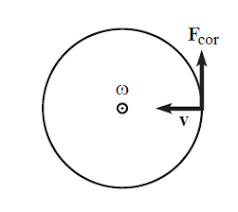The image above represent angular velocity.

To compute the angular velocity, one essential parameter is needed and its parameter is Number of Revolutions per Minute (N).

The formula for calculating angular velocity:

ω = 2πN / 60

Where;
ω = Angular velocity
N = Number of revolutions per minute

Let’s solve an example;
Find the Angular Velocity with a number of revolutions per minute as 60.

This implies that;
N = Number of revolutions per minute = 60

ω = 2πN / 60
ω = 2 x π x 24 / 60
ω = 150.816/ 60
ω = 2.5136

Therefore, the angular velocity is 2.5136 rad/s.

Calculating the Number of Revolutions per Minute when Angular Velocity is Given.

N = ω60 /

Where;
N = Number of revolutions per minute
ω = Angular velocity

Let’s solve an example;
With an angular velocity of 40. Find the number of revolutions per minute?

This implies that;
ω = Angular velocity = 40

N = ω60 /
N = 40 x 60 / 6.284
N = 2400 / 6.284
N = 381.9

Therefore, the number of revolutions per minute is 381.9 min.

## How to Calculate and Solve for Mass, Angular Velocity, Radius and Centrifugal Force of a Body | The Calculator Encyclopedia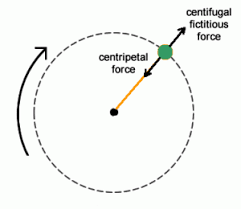The image above represents the centrifugal force.

To compute for the centrifugal force, three essential parameters are needed and these parameters are Mass of the body (m), Angular Velocity of the body (w) and Radius (r).

The formula for calculating the centrifugal force:

F = mω²r

Where:
F = Centrifugal Force
m = mass of the body
ω = angular velocity

Let’s solve an example;
Find the centrifugal force with mass of the body as 12, angular velocity as 32 and a radius of 8.

This implies that;
m = mass of the body = 12
ω = angular velocity = 32

F = mω²r
F = 12 x 32² x 8
F = 12 x 1024 x 8
F = 98304

Therefore, the centrifugal force is 98304 N.

Calculating the Mass of the body (m) when the Centrifugal Force, Angular Velocity and Radius is Given.

m = F / w2r

Where;
m = mass of the body
F = Centrifugal Force
ω = angular velocity

Let’s solve an example;
Find the mass of a body when centrifugal force is 140 with an angular velocity of 24 and a radius of 10.

This implies that;
F = Centrifugal Force = 140
ω = angular velocity = 24